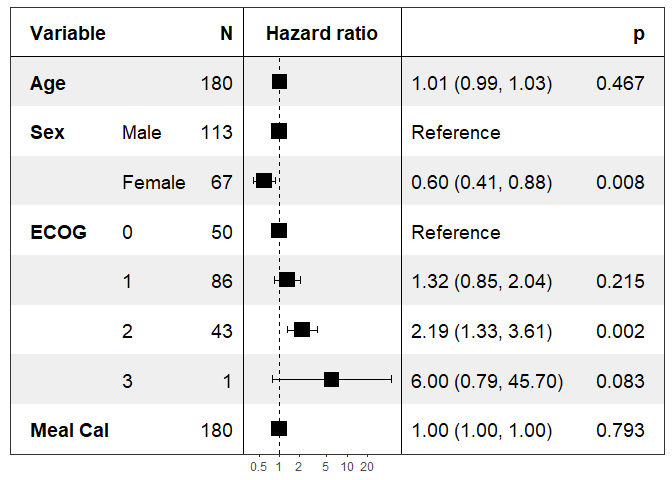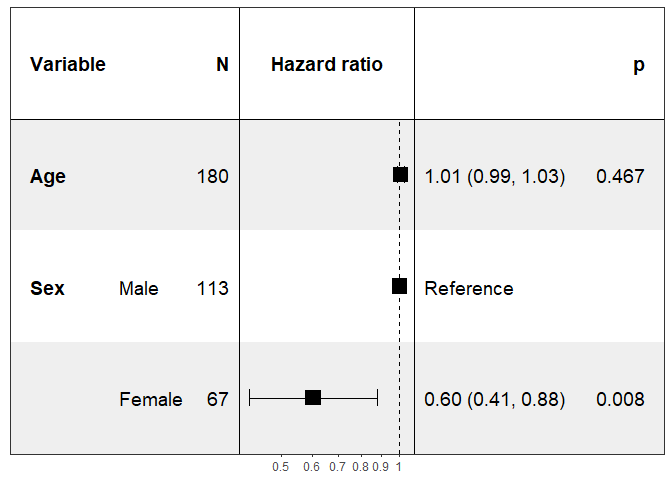# forestmodel

This is an R package to generate forest plots of the coefficients of models produced by `lm`, `glm`, `survival::coxph`, etc.

The main function is `forest_model`, with a helper function `default_forest_panels` to produce the necessary `panels` `data.frame`.

## Installation

The package can be installed using `install.packages`. It needs Hadley Wickham’s `broom`, `dplyr`, `gpplot2` and `lazyeval` packages.

Development takes place on the github repository https://github.com/NikNakk/forestmodel/.

## Installation

You can install the released version of forestmodel from CRAN with:

``install.packages("forestmodel")``

And the development version from GitHub with:

``````# install.packages("devtools")
devtools::install_github("NikNakk/forestmodel")``````

## Example

``````library(forestmodel)
library(survival)
library(dplyr)
pretty_lung <- lung %>%
transmute(time,
status,
Age = age,
Sex = factor(sex, labels = c("Male", "Female")),
ECOG = factor(lung\$ph.ecog),
`Meal Cal` = meal.cal)

print(forest_model(coxph(Surv(time, status) ~ ., pretty_lung)))``````Specifying the covariates to show:

``````print(forest_model(coxph(Surv(time, status) ~ ., pretty_lung),
covariates = c("Age", "Sex")))``````